Question

What is the net force exerted by these two charges on a third charge q3 = 53.0 nC placed between q1 and q2 at x3 = -1.120 m ? Your answer may be positive or negative, depending on the direction of the force. Express your answer numerically in newtons to three significant figures. q1 = -15.5 nC , is located at x1 = -1.695 m ;the second charge, q2 = 30.5 nC , is at the origin (x=0.0000).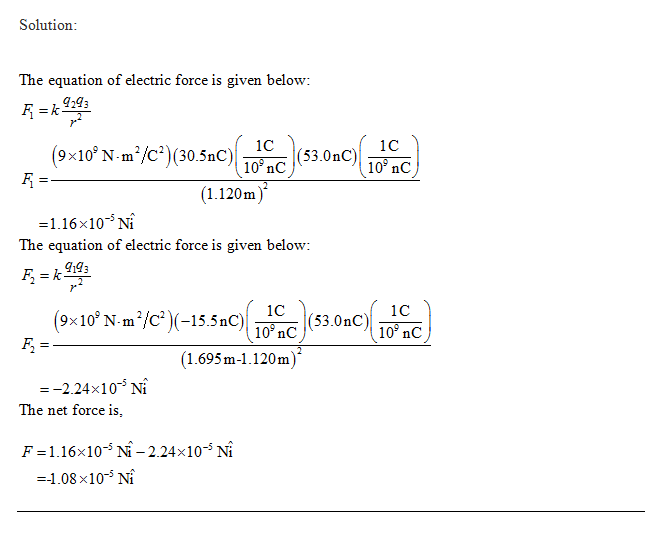#### Earn Coins

Coins can be redeemed for fabulous gifts.

Similar Homework Help Questions
• ### Consider two point charges located on the x axis: one charge, q1 = -15.5 nC ,...

Consider two point charges located on the x axis: one charge, q1 = -15.5 nC , is located at x1 = -1.695 m ; the second charge, q2 = 30.5 nC , is at the origin (x=0.0000). What is the net force exerted by these two charges on a third charge q3 = 53.0 nC placed between q1 and q2 at x3 = -1.120 m ? Your answer may be positive or negative, depending on the direction of the force.

• ### Consider two point charges located on the x axis: one charge, q1 = -15.5 nC ,...

Consider two point charges located on the x axis: one charge, q1 = -15.5 nC , is located at x1 = -1.695 m ; the second charge, q2 = 30.5 nC , is at the origin (x=0.0000). What is the net force exerted by these two charges on a third charge q3 = 53.0 nC placed between q1 and q2 at x3 = -1.120 m ? Your answer may be positive or negative, depending on the direction of the force.

• ### What is the net force exerted by these two charges on a third charge q3 =...

What is the net force exerted by these two charges on a third charge q3 = 46.5 nC placed between q1 and q2 at x3 = -1.205 m ? Your answer may be positive or negative, depending on the direction of the force. Express your answer numerically in newtons to three significant figures.   Consider two point charges located on the x axis: one charge, q1 = -10.0 nC , is located at x1 = -1.730 m ;the second charge, q2...

• ### What is the net force exerted by these two charges on a third charge q3 =...

What is the net force exerted by these two charges on a third charge q3 = 45.5 nC placed between q1 and q2 at x3 = -1.150 m ? Your answer may be positive or negative, depending on the direction of the force. Express your answer numerically in newtons to three significant figures. Coulomb's law for the magnitude of the force F between two particles with charges Q and Q′ separated by a distance d is |F|=K|QQ′|d2, where K=14πϵ0, and...

• ### What is the net force exerted by these two charges on a third charge q3 =...What is the net force exerted by these two charges on a third charge q3 = 49.0 nC placed between q1 and q2 at x3 = -1.245 m ? Your answer may be positive or negative, depending on the direction of the force. Express your answer numerically in newtons to three significant figures. Review | Constants | Periodic Table Coulomb's law for the magnitude of the force F between two particles with charges Q and Q' separated by a distance...

• ### What is the net force exerted by these two charges on a third charge q3 =...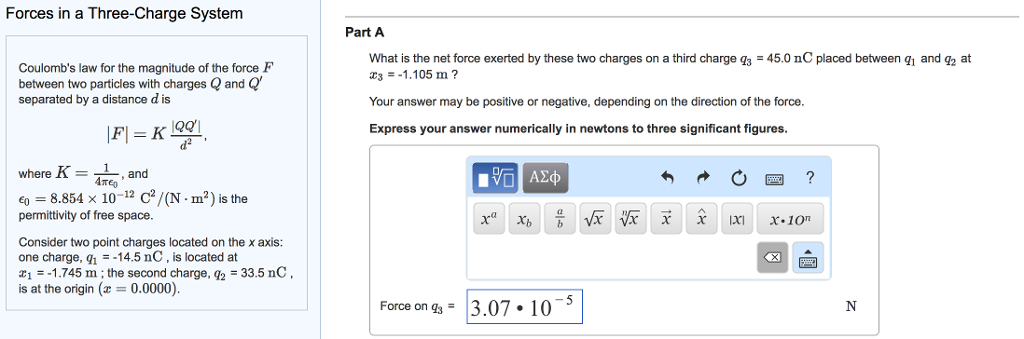What is the net force exerted by these two charges on a third charge q3 = 45.0 nC placed between q1 and q2 at x3 = -1.105 m ? Forces in a Three-Charge System Coulomb's law for the magnitude of the force F between two particles with charges Q and Q' separated by a distance d is where K 1 and ATeo eo 8.854 x 10 12 C/(N ma) s the permittivity of free space. Consider two point charges located...

• ### What is the net force exerted by these two charges on a third charge q3 =...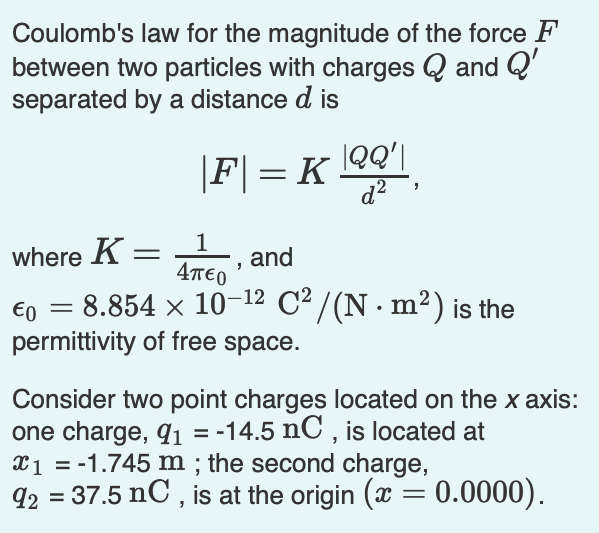What is the net force exerted by these two charges on a third charge q3 = 49.0 nC placed between q1 and q2 at x3 = -1.070 m ? Coulomb's law for the magnitude of the force F between two particles with charges Q and Q separated by a distance d is where , and A 8.854x 10-12 C2/(N. m2) is the permittivity of free space Consider two point charges located on the x axis: one charge, 1-14.5 nC, is...

• ### What is the net force exerted by these two charges on a third charge q3 =...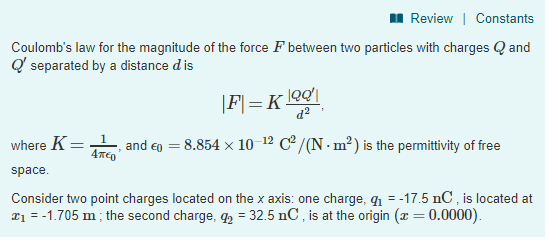What is the net force exerted by these two charges on a third charge q3 = 46.0 nC placed between q1 and q2 at x3 = -1.235 m ? Your answer may be positive or negative, depending on the direction of the force. Force on q3 q3q_3 = Review | Constants Coulomb's law for the magnitude of the force F between two particles with charges and separated by a distance d is where K-L and印= 8.854 × 10 i2 C2/(N-m2)...

• ### What is the net force exerted by these two charges on a thirdcharge q3 =...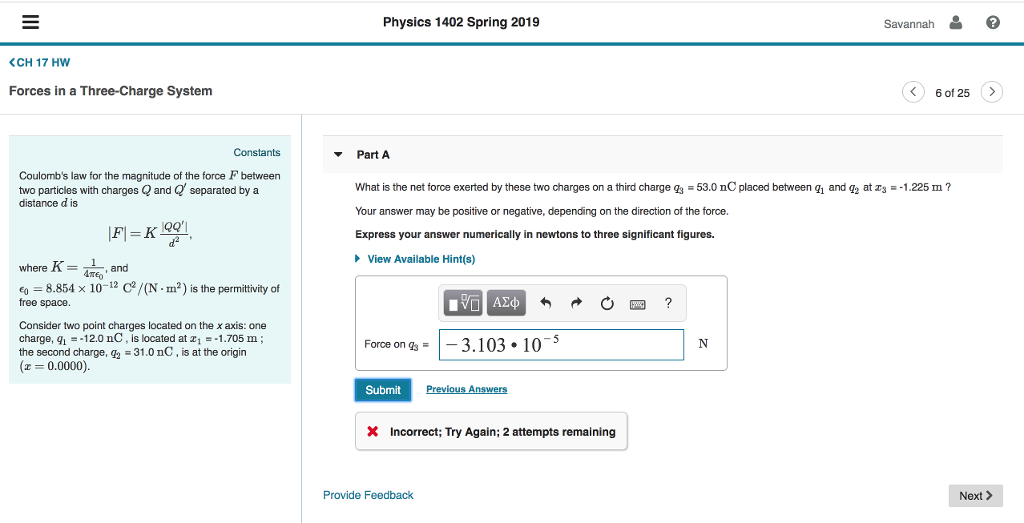What is the net force exerted by these two charges on a third charge q3 = 53.0 nC placed between q1 and q2 at x3 = -1.225 m ? Your answer may be positive or negative, depending on the direction of the force.

• ### Coulomb's law for the magnitude of the force F between two particles with charges Q and...

Coulomb's law for the magnitude of the force F between two particles with charges Q and Q′ separated by a distance d is |F|=K|QQ′|d2, where K=14πϵ0, and ϵ0=8.854×10−12C2/(N⋅m2) is the permittivity of free space. Consider two point charges located on the x axis: one charge, q1 = -16.0 nC , is located at x1 = -1.675 m ; the second charge, q2 = 37.0 nC , is at the origin (x=0.0000). Part A What is the net force exerted by...